# 1. Model Inputs & Meshing

The initial model inputs and meshing are key to achieving accurate simulation results. We will look at these aspects of the model for both modes of operation.

## Variables & Parameterization

Parameterizing and using variables in a model allows changes to be applied to your model very quickly and intuitively. We should always use variables where possible to make model generation process as simple as possible.

Typical variables in model file include:

• All useful dimensions to control geometry
• Mesh Setting Variables
• Keypoints in XYZ space and IJK grid space
• Model ExecutionVariables

### Designer Mode

All the required variables for a model will be accesible through the Model Tree and set within the Properties window: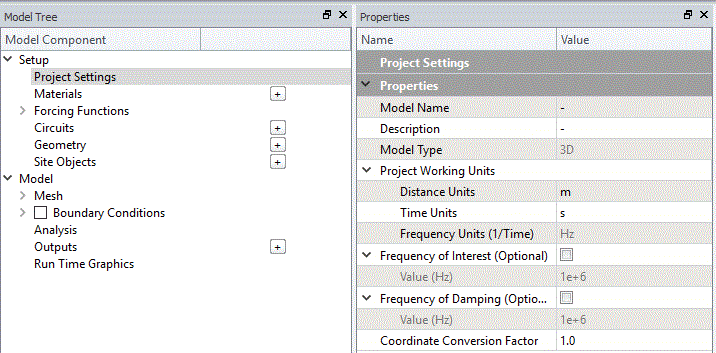### Analyst Mode

All variables must be created using the symb command:

`symb freqint = 433.e6 /* setting a variable for frequency of interest`

## Mesh Settings

Meshing is one of the key components to obtaining accurate results. The elements that are used to construct a mesh must take into account a number of aspects to be able to discretise stress gradients accurately within the model. The following parameters are typically used to determine the required element size which we commonly refer to as box:

1. Frequency of Interest
• Typical variable name - freqint
• Set to the centre of the frequency band that you are interested in
2. Slowest wave velocity in the model
• Typical variable name - vel
• Generally set to the lowest shear wave velocity in the model
3. Shortest wavelength in the model
• Typical variable name - wavelength
• Calculation of the shortest wavelength based on vel and freqint
4. Number of element to discretise a wavelength
• Typical variable name - nepw
• Minimum value of 15 elements per wavelength is recommended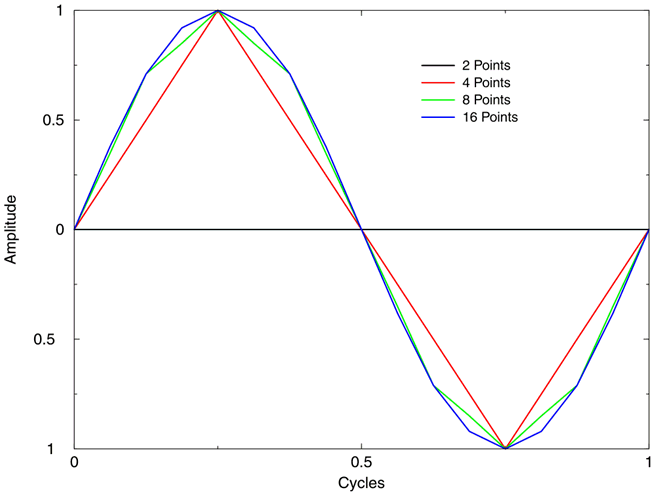5. Size of an element
• Typical variable name - box
• Calculated from the wavelength divided by number of elements per wavelength

### Designer Mode

All required information is extracted from the Time Function frequency input, slowest material velocity from the project material list and a default value of 15 number of elements per wavelength is used. All calculations are performed automatically based on the mentioned inputs. Note that, any of these values can be overridden by selecting the checkbox for each of the parameters: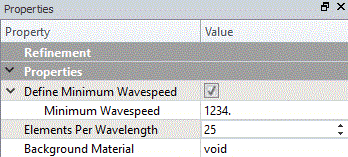### Analyst Mode

The following symb variables are created and calculations are performed to find the element size:

`c frequency and meshsymb freqint = 1e6 /* frequency of interestsymb freqdamp = \$freqint /* set damping frequency to frequency of operationsymb vel = 1500 /* minimum material velocity in modelsymb wavelength = \$vel / \$freqint /* calculate smallest wavelength to discretisedsymb nepw = 15 /* number of elements per wavelengthsymb box = \$wavelength / \$nepw /* calculate element size for meshing`

## Keypoint Generation and Grid Setup

Keypoints are critical points in space that will be used to accurately map out the dimensions of your geometry. For geometries where physical dimensions has as direct effect on resonant behaviour such as the thickness of a PZT plate then, we must ensure that the dimension is represented accurately.

Keypoints are simple variables that hold an XYZ location value or IJK node value when tied to the mesh grid. When we have all the XYZ keypoints in a model, we can calculate how many elements are required in the model and generate the grid of nodes which will form our mesh. By using keypoints when generating the mesh, it will stipulate that a plane of nodes must lie on the XYZ keypoint dimension creating a non uniform standard partitioned grid: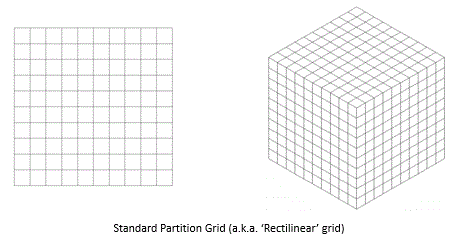No KeyPoints Used in Mesh Generation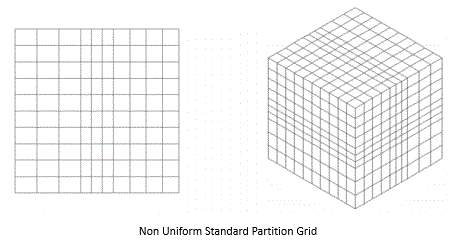KeyPoints Used in Mesh GenerationTip: User should always use keypoints to ensure geometries are correctly and accurately defined with OnScale.

### Designer Mode

All keypoint generation is handled graphically by clicking at XYZ locations on the CAD geometry. The edge detection algorithm makes it easy to define keypoints at vital locations on the CAD. Setting a keypoint will ensure edges and features are at the intended dimension when designed in the external CAD package: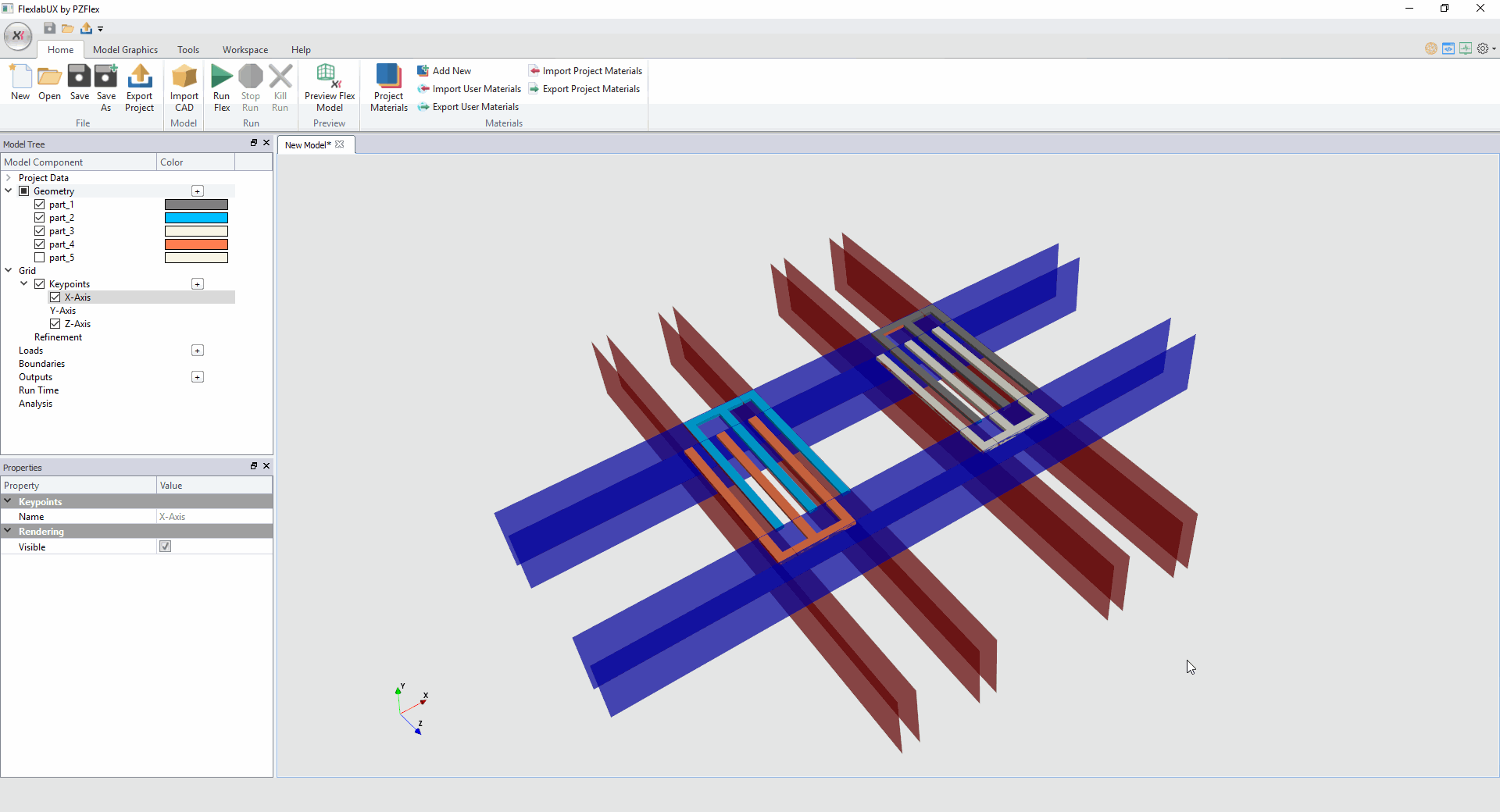### Analyst Mode

We use a range of commands to set up both the keypoints and the grid:

• symb #keycord - quick method of generating XYZ keypoints
• symb #keyindx - quick method of generating IJK keypoints
• grid command - creates Standard Partition Grid
• geom command - ties in XYZ keypoints to IJK nodal keypoints to create the Non Uniform Partitioned Grid
```c create x-y-z keypoints to accurately model dimensionssymb #keycord x 1 0.0 \$dimension1 \$dimension2 \$dimension3 /* etc...symb #get { idx } rootmax x /* store last keypoint indice in variable 'idx'
symb #keycord y 1 0.0 \$dimension4 \$dimension5 \$dimension6 /* etc...symb #get { jdx } rootmax y /* store last keypoint indice in variable 'jdx'
c create i-j-k keypointssymb #keyindx i 1 \$idx 1 \$box 1 /* calculate number of nodes required in the i directionsymb #keyindx j 1 \$jdx 1 \$box 1 /* calculate number of nodes required in the j direction
symb indgrd = \$i\$idx /* last node number in i directionsymb jndgrd = \$j\$jdx /* last node number in j direction
c create gridgrid \$indgrd \$jndgrd axiy /* generate grid of nodes and apply axisymmetric condition to model grid
c map physical geometry to grid of nodesgeom keypnt \$idx \$jdx /* tie in all xy keypoints (physical dimensions) to ij keypoints(nodes)```